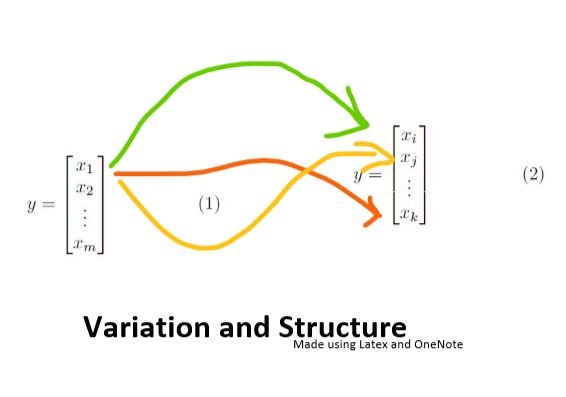# Studying abstract mathematical structures using data, ML, AICategory
Facilitating Research
Description

The notion of isomorphism of a list

Consider a list [3, 4, 5, 6, 7, 8, 9, 16, 25, 36, 49, 81]. Let’s say that a machine learning algorithm identifies a pattern and gives
a substructure to the list. [3, 4, 5, 6, 7, 8] joined with [9, 16, 25, 36, 49, 81] where an element in the first list could be associated to an element in the second list via squaring. This defines a map from [3, 4, 5, 6, 7, 8, 9, 16, 25, 36, 49, 81] to itself hence an Isomorphism to itself. Another algorithm may identify a different substructure. [3, 16, 5, 36, 7, 64] joined with [9, 4, 25, 6, 49, 8] where an odd element is associated to its square in the second list and an even element in the first list is identified to its square root in the second list. This is another isomorphism of the list [3, 4, 5, 6, 7, 8, 9, 16, 25, 36, 49, 81]. One could approximate the notion of Permutation action of a Symmetric group on a list by finding out isomorphism from the set to itself, by producing different maps. The longer the list gets the better the approximation could get. Naively it seems so but probably would require academic papers on the subjects to study behaviors of algorithms and data structures in approximating a symmetric group.

Representing abstract mathematical structures with data

Abstract mathematical structures can be challenging to represent on a computer. If one were to represent a group of 10 elements, one would have to define inverse of each element and operation of every pair of elements. And if one were to approximate continuous mathematical objects like a Lie Group with a data structure by brute force, it would quickly get out of hands and become very messy.

One way of implementing abstract mathematical structures could be using structuring of a random or predetermined data as discussed above. Consider a path joining two points on the boundary of a rectangle (a generic ‘find your way through the maze’ game scenario), allowing desired flexibility on the length of the path. Now let’s give an algorithm 10,000 boxes (an empty list of capacity 10,000 items) and 5000 blocks to be filled in the array. The transition between different states of filling the box with blocks in different configurations could define an ‘evolution structure’. One could use that to represent a vibrating string randomly or otherwise. One could use a quantum computing simulator to allow superposition of states of boxes being filled or empty. Could this try to simulate the behavior of a quantum string?

Another notion that’s interesting to me is the idea of a manifold. I assume that the easiest way to define a manifold in a computer is through the means of charts connection. Picture multiple path through the ‘maze’ in the previous example.

But how could such structures be useful?

Possible investigations of use

These ideas could help understand some problems and ideas in pure mathematics through this ‘perturbative approach’. Sophus Lie was motivated to model the symmetries of differential equations by Lie Groups. Many partial differential equations are solved numerically using different algorithms. Solving partial differential equations is a pretty big deal but not all kinds of partial differential equations are solved with numbers. The existence of solutions to partial differential equations are studied in pure mathematics and sometimes open questions. Could representing a Lie Group on a computer help solve some partial differential equations numerically? Likewise, structures in algebraic geometry could help solve algebraic equations. One could perhaps study the nature of the quantum least action principle for gravity, an exponential of integral.

One could also be able to use these notions for more concrete purposes. For example the isomorphism from a ‘seemingly random’ or random list could output a different map each time providing a new key each time for security on an online application. Given two images, what is the possible modification on the first image that can be done to obtain the second image. One could in this manner compare two objects of data. Could one describe possible ways of breaking a security algorithm from the supposedly secure state of the program, and help understand security breaches? Or this could be used to study the time order in which an algorithm can be reduced to a different algorithm.

Summary

The idea behind this project is to study some ways to define abstract mathematical objects with the use of data, machine learning and artificial intelligence and understand whether they are useful and efficient or just messy. The first notion would be maps from a list to itself which could potentially provide a lot of functionality.

Any suggestions and ideas is welcome!

Weekend Goals

To study the  isomorphisms from a random or otherwise list to itself using data, Machine Learning and Artificial Intelligence and understand whether it can be useful  in  computations and algorithms.

Skills being sought
• Some experience of using Programming Language. I know some Python and a bit of C but one could use functionalities from other programming languages.
• Data: Using Pandas, Networkx modules from Python could be useful to begin with.
• Machine Learning: Using k-nearest neighbor, Linear Regression and Classifier, Forest Regression and Classifier,  could be useful  to begin with to study structures in a list.
• Artificial Intelligence:  Something like reordering of lists could be used to study search algorithms.
References and background material

I have just started studying https://www.edx.org/course/cs50s-introduction-to-artificial-intelligence-with-python  and learned a bit about Search in week 0 of the course.

I recently sort of completed studying https://www.edx.org/course/using-python-for-research#:~:text=Introducti… , where I learned about pandas, scikitlearn, networkx modules of python.

Previously I learned a bit about web application https://www.edx.org/course/cs50s-introduction-to-computer-science 

Some knowledge about groups could be useful https://en.wikipedia.org/wiki/Group_(mathematics)